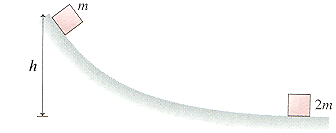# Problem: A package of mass m is released from rest at a warehouse loading dock and slides down a h = 3.6 m high frictionless chute to a waiting truck. Unfortunately, the truck driver went on a break without having removed the previous package, of mass 2m, from the bottom of the chute.(a) Suppose the packages stick together. What is their common speed after the collision?m/s(b) Suppose the collision between the packages is elastic. To what height does the package of mass m rebound?cm

###### FREE Expert Solution

(a)

Conservation of energy:

P.E = K.E

$\begin{array}{rcl}\mathbf{m}\mathbf{g}\mathbf{h}& \mathbf{=}& \frac{\mathbf{1}}{\mathbf{2}}\mathbf{m}{{\mathbf{v}}_{\mathbf{0}}}^{\mathbf{2}}\\ {\mathbf{v}}_{\mathbf{0}}& \mathbf{=}& \sqrt{\mathbf{2}\mathbf{g}\mathbf{h}}\\ & \mathbf{=}& \sqrt{\mathbf{\left(}\mathbf{2}\mathbf{\right)}\mathbf{\left(}\mathbf{9}\mathbf{.}\mathbf{81}\mathbf{\right)}\mathbf{\left(}\mathbf{3}\mathbf{.}\mathbf{6}\mathbf{\right)}}\end{array}$

v0 = 8.4 m/s

Conservation of momentum:

98% (313 ratings)###### Problem Details

A package of mass m is released from rest at a warehouse loading dock and slides down a h = 3.6 m high frictionless chute to a waiting truck. Unfortunately, the truck driver went on a break without having removed the previous package, of mass 2m, from the bottom of the chute.(a) Suppose the packages stick together. What is their common speed after the collision?

m/s

(b) Suppose the collision between the packages is elastic. To what height does the package of mass m rebound?

cm